﻿ 1, 2, 3, code ! - Cycle 3 activities - Lesson 1.3. How to code information in binary | Le site de la Fondation La main à la pâte

# 1, 2, 3, code ! - Cycle 3 activities - Lesson 1.3. How to code information in binary

 Summary Students must now use only two symbols (0 and 1) to transmit messages. They explore the ways of encoding different information (the four cardinal directions – North/South/East/West, the seven days of the week, etc.) by combining 0s and 1s. This is binary coding. Key ideas (see Conceptual scenario) “Information” A computer represents all information using a code with only two symbols, 0 and 1, called bits: this is binary code. Binary code makes it possible to represent all kinds of data, especially numbers and letters. The more bits are combined, the greater the variety of elements can be represented. Inquiry-based methods Experimentation Equipment For each student Glossary List of elements, bit, binary code Duration 1hour 30 minutes

## Introductory question

The teacher explains to the class that electronic instruments cannot directly transmit numbers: they transmit flows of light or electrical signals. These signals have only two states of being: NO (no signal) or YES (signal), also referred to as 0 and 1. Students must revise their coding system with this new limitation.

The question is: How do you encode information by using only 0s and 1s?

## Activity: Find an encoding strategy using only 0s and 1s (in pairs and as a class)

The teacher reminds the students that the rover can be operated by mission control using the four words North/South/East/West. They ask the students to work in pairs to find a way to transmit these four words using only 0s and 1s.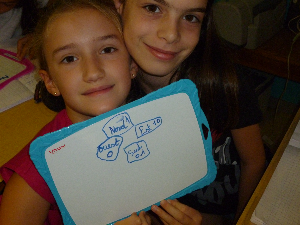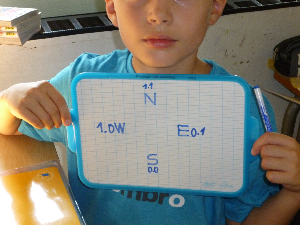After a few minutes, the groups share their solutions (see Teaching notes below).

The class discusses the suggestions and observes that those that work all use groupings of 0s and 1s.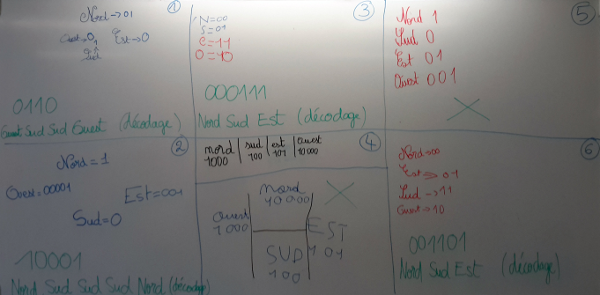Remind the group about the importance fo encoding each piece of information using the same number of numbers for decoding. Fifth Grade class, Christelle Crusberg, Champigny-sur-Marne).

Teaching note:

• A first solution could be to replace:

"North" with "00"

"South" with "11"

"East" with "01"

"West" with "10"

For this type of suggestion, each cardinal direction is coded with a pair of 0s or 1s. All combinations of two 0s and 1s are used. It is important to tell students that each group may have a different cardinal direction linked to a given number pair: all combinations are valid.

• A second solution could be to replace:

"North" with "1000"

"South" with "0100"

"East" with "0010"

"West" with "0001"

For this type of suggestion, each cardinal direction is coded with a series of four 0s or 1s. Only certain combinations of four 0s and 1s are used (for example, the combination 0000 is not used).

• A third solution could be to replace:

•  « North » with: « South » with: « East » with: « West » with: 1 0 0 0 0 0 0 0 0 1 1 0 0 1 0 0
For this type of suggestion, the teacher notes that it is a good idea, but that the 0s and 1s cannot be transmitted unless they are next to each other, so they cannot be spread out. This third suggestion is the equivalent of suggestion 2: for each cardinal direction, four 0s or 1s are transmitted, and only one is a 1. There are four possible positions for the 1, one for each cardinal direction.

## Exercise: Choosing the number of 0s and 1s to combine in order to code the days of the week (in groups)

The teacher tells the students that all messages exchanged between the base and the rover have dates. They need to be able to indicate the day of the week (among other information) using 0s and 1s. The teacher asks the students to suggest a way to code the seven days of the week using the fewest combinations of 0s and 1s possible.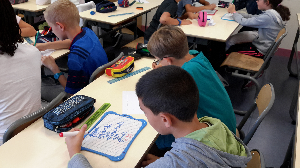Fifth Grade class, Christelle Crusberg, Champigny-sur-Marne

## Group discussion

The group discussion shows that combining three 0s or 1s (as below) is one way (of many equivalent possibilities) to accomplish the task:

000 for Monday

001 for Tuesday

010 for Wednesday

011 for Thursday

100 for Friday

101 for Saturday

110 for Sunday

There is even another combination (111) that has not been used.

The teacher guides the students in forming an oral conclusion using Handout  30 projected and handed out to all students:

We can code the elements of a list using a series of 0s or 1s, also called bits (a contraction of "binary digit").

• With a single bit, we can code all elements on a list that has no more than two elements (black/white or on/off are examples of lists with two elements): we link the 0 to one element and the 1 to the other element on the list (the class writes down list examples with two elements on Handout 30).
• With two combined bits, we can code all elements on a list that has no more than four elements (e.g., North/South/East/West) because there are four different ways to combine pairs of 0s and 1s: "00," "01," "10" and "11" (the class writes down examples of lists than can be coded using 2 bits but not 1 bit on Handout 30: these lists have three or four elements).
• With three combined bits, we can code all elements on a list that has no more than eight elements (e.g., the seven days of the week), because the only eight possible combinations of 0s and 1s are: "000," "001," "010," "011," "100," "101," "110" and "111" (the class writes down examples of lists than can be coded using 3 bits but not 2 bits on Handout 30: these lists have five to eight elements).
• The more bits are combined, the more different elements can be represented: a maximum of 16 elements combining 4 bits, 32 elements combining 5 bits, 64 elements combining 6 bits, etc.

Each student writes down on Handout  30 a list that can be coded using 4 bits but not 3 bits (the list should have between nine and 16 elements). Students may suggest the 12 months of the year, the 10 figures 0 through 9, the 12 hours on a clock, the 10 fingers of the hands, the names of nine of their cousins, 16 farm animals, etc.

## Challenge (in pairs)

The teacher asks the students to find how many bits they need to combine to be able to code the 26 letters of the alphabet.

After a few minutes, the group discussion will show that four bits is not enough to code the 26 letters (four bits = maximum coding of 16 elements: 2x2x2x2=16), but five bits is (maximum coding of 32 elements: 2x2x2x2x2=32).

## Conclusion and lesson recap activity

The class summarizes together what they learned in this lesson:

• We can encode the elements on a list using a series of 0s and 1s, also called bits. This is what is called "binary code".
• The more bits are combined, the more different elements can be represented: A maximum of two elements with one bit, a maximum of 2x2=4 elements by combining two bits, 2x2x2=8 by combining three bits, 2x2x2x2=16 elements by combining four bits, 2x2x2x2x2=32 bits by combining five bits, 2x2x2x2x2x2x2x2=256 bits by combining eight bits, etc.
• Binary code makes it possible to represent all kinds of data, especially numbers and letters.
• A computer represents all types of information using binary code.

The students write down these conclusions in their science notebook while the teacher adds this information to the "Defining computer science" poster.

## Further study

An online exercise to reappropriate the concept of binary enumeration.

Un exercice en ligne permet de se réapproprier le concept d'énumération binaire.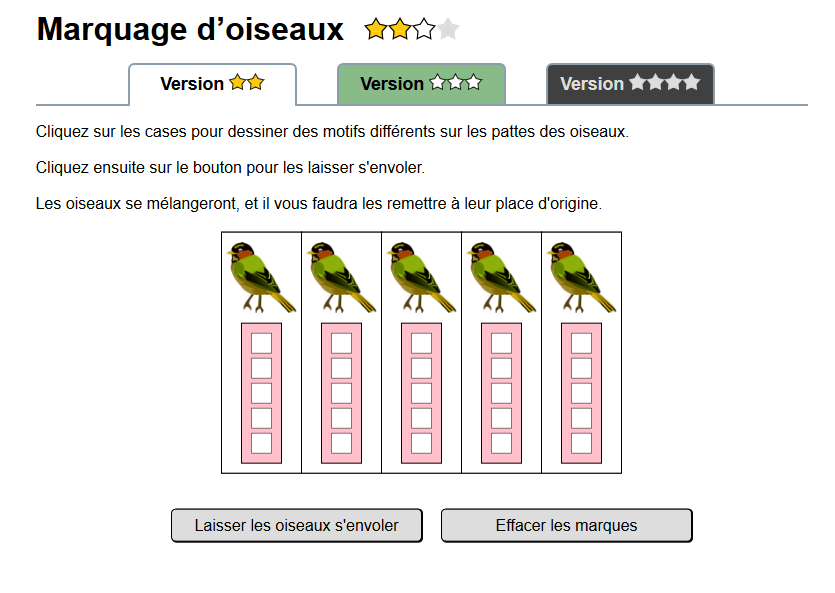## Further study (for Cycle 4)

In Cycle 4, additional attention can be paid to how to easily find all combinations of 0s and 1s for a given number of bits. For example, if you ask students to find the 32 possible combinations of five bits, they will realize it is very difficult to find them all without repeating some of them.

The teacher can suggest a recursive approach:

• With just one bit, the list is easy: 0, 1.
• For two bits, simply copy the one-bit list twice: once adding a 0 at the start, once adding a 1 at the start, to get 00, 01, 10, 11.
• For three bits, the process is the same. Copy the two-bit list twice: once adding a 0 at the start, once adding a 1 at the start to get 000, 001, 010, 011, 100, 101, 110, 111. The process is the same for four bits, five bits, etc.

 << Lesson 1.2 Sequence I Lesson 1.4 >>

Aucun résultats